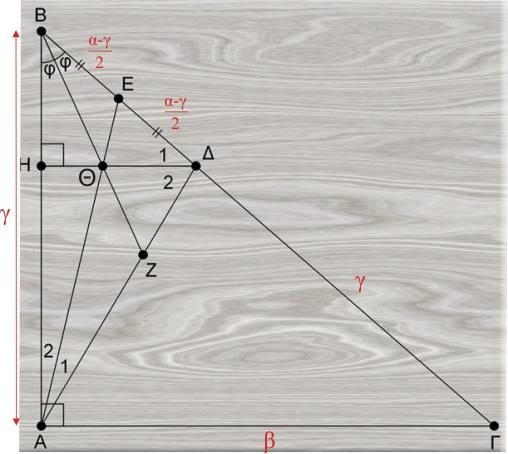# Concurrence in Right Triangle

### Problem### Solution 1

We shall make use of Ceva's theorem (or rather its converse): let $CZ\,$ be the angle bisector, $BE\,$ the median, $DH\,$ the altitude. We need to show that

$\displaystyle \frac{CH}{HA}\cdot\frac{AZ}{ZD}\cdot\frac{DE}{EC}=1.$

Since $DE=EC,\,$ remains it to show that $\displaystyle \frac{CH}{HA}=\frac{ZD}{AZ}=\frac{CD}{AC},\,$ by a property of angle bisector. We have

$\displaystyle \frac{AC-HA}{HA}=\frac{CH}{HA}=\frac{CD}{AC}=\frac{BC-BD}{AC}=\frac{BC-AC}{AC},$

or, $\displaystyle \frac{AC}{HA}=\frac{BC}{AC}=\frac{BC}{BD}\,$ which is true by Thales' theorem because $DH\parallel AB.$

### Solution 2Let $K\,$ be the intersection point of the median $AE\,$ of $\Delta ABD\,$ and the angle bisector of $\angle B.\,$ From the angle bisector property in $\Delta ABD,\,$ $\displaystyle \frac{EK}{KA} = \frac{BE}{BA}\overset{BE= ED, BA=DC}{=}\frac{ED}{DC},\,$ from which, by the converse of Thales' theorem, $\displaystyle KD\parallel AC\overset{AC_\perp AB}{=>} KD\parallel AB,\,$ and the equivalent problem has been proved.

### Solution 3Let $ABQC\,$ be a rectangle and $ABTD\,$ be a parallelogram. Then obviously $CQTD\,$ is rhombus and because $(T.BSDQ)\,$ is a harmonic system of points, as $TQ\parallel BD\,$ and $BE=ED,\,$ we also have that $(D.BSTQ)\,$ is also a harmonic system of points; therefore $DS\,$ is angle bisector of $\Delta BDT\,$ (because $DQ\,$ is external angle bisector). Thus, due to symmetry, $BZ\,$ is also angle bisector of $\Delta BAD.$

### Solution 4Let $AE\,$ be the median and $BZ\,$ the angle bisector of $\Delta ABD,\,$ interecting in $K.\,$ We draw $D\Theta.\,$ Its extension cuts $AB\,$ in point $H.$

In $\Delta BEA,\,$ from the Angle Bisector Property, $\displaystyle \frac{E\Theta}{\Theta A} = \frac{BE}{BA}=\frac{a-c}{2c} = \frac{ED}{DC}.$

Thus, by converse of Thales Theorem we take $D\Theta\parallel AC,\,$ therefore $\Delta H\,$ is altitude in $\Delta ADB.$

P.S. Symbols different from those picture:

\displaystyle \begin{align} &\Gamma= C,\\ &\Delta = D,\\ &\gamma = c [= (AB)]\\ &\alpha= a [= (BC)]\\ &\beta = b [= (AC)]. \end{align}

### Solution 5Let $AZ\,$ be an angle bisector and $DE\,$ the altitude in $\Delta BAD\,$ with $K\,$ their intersection point. $AK\,$ cuts $BD\,$ in point $\Theta.\,$ We shall prove that $\Theta\,$ is the midpoint of $BD.$

Let $KM=KD\,(1).$

Since $ED\parallel AC,\,$ $\displaystyle\frac{EB}{EA}=\frac{BD}{CD}\,(2)\,$

and from $\displaystyle \frac{BD}{BE}=\frac{BC}{BA},\,$ $\displaystyle \frac{BD}{DE}=\frac{BC}{DC}\,(3).\,$

In $\Delta BED,\,$ $AK\,$ is an angle bisector. and from the related property we have $\displaystyle \frac{KD}{KE}=\frac{BD}{BE},\,$ implying (via (1),(3)) that

\displaystyle\begin{align}&\frac{KM}{KE}=\frac{BC}{DC}&\Longrightarrow\\ &\frac{KM-KE}{KE}=\frac{BD-DC}{DC}&\Longrightarrow\\ &\frac{ME}{KE}=\frac{DB}{DC}\,\text{(due to (2))}&\Longrightarrow\\ &\frac{ME}{KE}=\frac{AB}{EA}&\Longrightarrow\\ &MB\parallel AK. \end{align}

Thus, in $\Delta MBD\,$ $K\,$ is the midpoint of side $MD\,$and $K\Theta\parallel MB.\,$ Therefore, $\Theta\,$ is the midpoint if $BD.$

Symbols different from those in the diagram:

\begin{align} \Gamma = C,\\ \Delta = D. \end{align}

### Acknowledgment

The problem has been posted at the Οι Ρομαντικοι της Γεωμετριας (Romantics of Geometry) facebook group. Takis Chronopoulos has generously volunteered to translate the posted proofs into English. Solution 2 is by Stathis Koutras; Vaggelis Stamatiadis and, independently, by Kostas Dortsios posted proofs same as in Solution 1; Solution 3 is by Andreas Varverakis; Solution 4 is by George Rizos; Solution 5 is by George Rodopoulos.

70356000